# Basic Course of Thermo-Fluid Analysis 09: Chapter 4 Basics of Heat - 4.1 Temperature and heat, 4.2 Buoyancy, 4.3 Natural convection and forced convection

#### Chapter 4 Basics of Heat I

This chapter describes four aspects of heat: temperature and heat, buoyancy, natural and forced convections, and heat transfer. All units are SI units.

#### 4.1 Temperature and heat

Temperature is the numerical measure of hot and cold. Its units are [°C] or [K]. Celsius temperature uses the unit [°C] while absolute temperature uses [K]. One degree in both temperature scales represents the same temperature difference. However, the base temperatures for the two scales are different. The relationship between the two temperature scales is expressed by the following equation: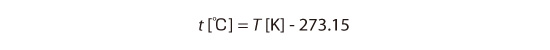A temperature of 0 °C is equal to 273.15 K.
An object can possess internal energy which is represented by its temperature. Heat is one form of the internal energy. When heat flows into an object, its temperature rises. When heat flows out of an object, its temperature decreases. The amount of heat is expressed in joules [J]. The amount of heat per unit time is expressed in watts [W]. Consider a room in the winter. As shown in Figure 4.1, when the heater is turned on, the room temperature rises. The heat from the heater is transferred to the air in the room, and the room air temperature rises.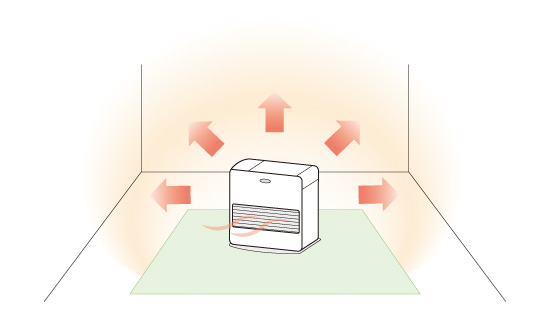Figure 4.1: Heat transfer when the heater is on

Figure 4.2 shows when the heater is turned off, the room temperature decreases. This is because heat is transferred from the air inside the room to the outside air causing the room air temperature to decrease.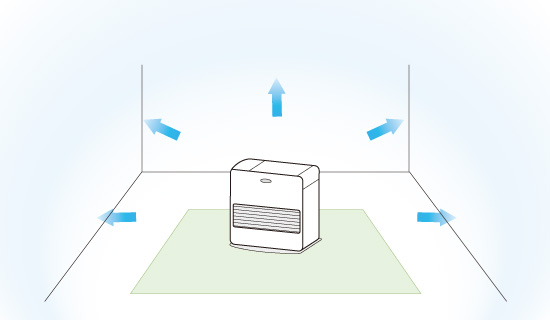Figure 4.1: Heat transfer when the heater is off

Heat transfers from objects with a higher temperature to objects with a lower temperature. When two objects with different temperatures are in contact with each other, their temperatures eventually become equal.

#### 4.2 Buoyancy

When the temperature of a fluid increases, the molecules (atoms) within the fluid move around more actively. As a result, the volume of the fluid increases and the density of the fluid decreases. When a fluid is heated, a force opposite of gravity occurs due to the density difference. This force is called buoyancy. A hot-air balloon, as shown in Figure 4.3, is a typical example of buoyancy.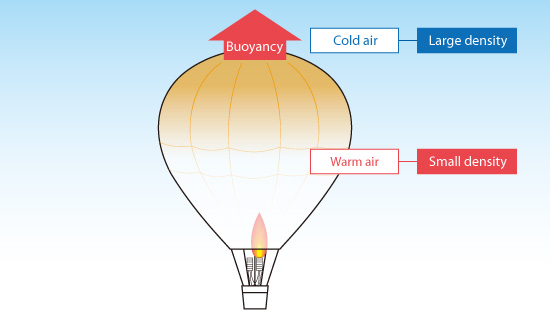Figure 4.3: Principle of hot-air balloon

Buoyancy of a compressible fluid can be treated in a straightforward manner because the volume is inversely proportional to the temperature. On the other hand, the volume change of an incompressible fluid is difficult to quantify. Because of this, the buoyancy of an incompressible fluid is approximated using a force that is proportional to temperature difference. This approximation is called the Boussinesq approximation. The error caused by the Boussinesq approximation is large when the temperature difference is very large.

#### 4.3 Natural convection and forced convection

The flow of fluid is classified in two ways depending on what is driving the flow. The two methods are: natural convection and forced convection.

With natural convection, heat flows without any assistance from external sources, e.g. a fan or pump. Natural convection is driven by buoyancy which is caused by differences in the fluid temperature. On the other hand, with forced convection, heat flow is caused by an external source such as a fan or pump, which moves the fluid. In (a) of Figure 4.4, a container of water is heated, and the heated water starts rising from the bottom of the container to the top of the container due to buoyancy. This is natural convection. On the other hand, as shown in (b) of Figure 4.4, when the water in the container is stirred with a stick, a flow is created which is caused by an external factor. This is an example of forced convection.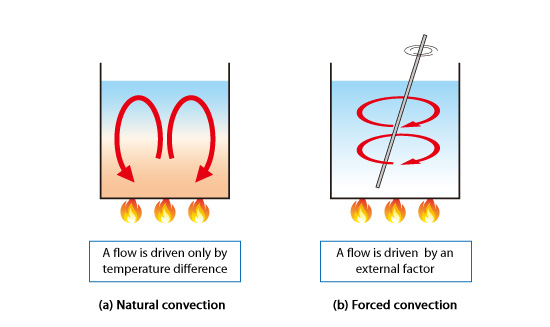Figure 4.4: Natural convection and forced convection

For forced convection, the buoyancy effect is small compared to the inertia force. This is a characteristic of flows driven by an external force such as a fan. Because the buoyancy effect is so small, it does not need to be considered in some analysis cases. However, in a flow with only natural convection or a flow with equal natural and forced convections, buoyancy effect cannot be ignored.